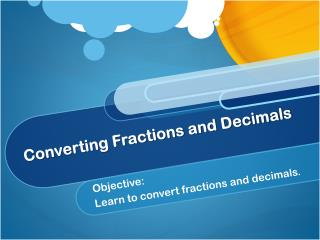DownloadDownload PresentationConverting Fractions and Decimals

# Converting Fractions and Decimals

Télécharger la présentation## Converting Fractions and Decimals

- - - - - - - - - - - - - - - - - - - - - - - - - - - E N D - - - - - - - - - - - - - - - - - - - - - - - - - - -
##### Presentation Transcript

1. Converting Fractions and Decimals Objective: Learn to convert fractions and decimals.

2. Fractions and decimals are different ways of writing the same quantity. • To convert from a decimal to a fraction, think of the place value the last number holds. • For example: 0.7 reads seven tenths. 0.7 = 7 seven 10 tenths • After converting a decimal to a fraction, you have to write it in simplest form is possible.

3. For Example: • 0.5 = • 0.23 = • 2. 66 =

4. To convert a fraction to a decimal… • To convert from a fraction to a decimal, you have to divide the numerator by the denominator. • The decimal you obtain will terminate or repeat. • A terminating decimal ends. • For example: 3 8

5. A repeating decimal repeats a pattern of digits. • For example: 3 11 You can use bar notation to indicate that a number pattern repeats indefinitely. A bar is written only over the digits that repeat.

6. For example: • 3 4 25 7 • 6 1 = 6 + 1 = 6 + 0.50 = 6.50 2 2

7. Homework: Write each decimal as a fraction in simplest form. 0.2 0.9 0.55 0.34 0. 66 Write each fraction or mixed number as a decimal. Write if it is terminating or repeating. Use bar notation if the decimal is repeating. 6. 4 10. 2 5 5 7. 5 16 8 . 8 11 9. 5 1/3